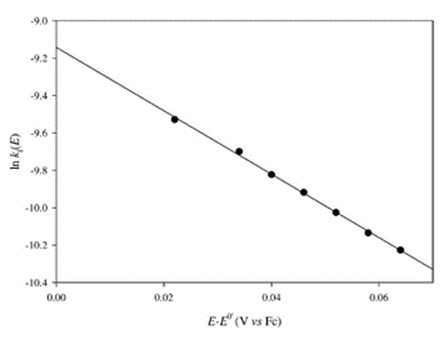# Electrochemistry of Room-Temperature/ Articles about substances designated as ionic liquids have begun to appear with increasing regularity in chemistry journals around the world, страница 15

il = 0.62nFAC*D2/3υ-1/6ω1/2                               (13)

where υ is the kinematic viscosity of the solution (cm2 s-1) and all other symbols have their usual meaning. An example of a Levich plot is depicted in the inset of Fig. 8. In the absence of complications, this plot should be a straight line passing through the origin. The slope of this plot can be used to estimate DO or DR, depending whether the oxidized or reduced form of the solute, respectively, is present in the solution at the beginning of the experiment. Following the U.S. sign convention for current, the relationship between the potential and current for a RDE voltammetric wave in the case of a reversible electrode reaction, where both the oxidized and reduced species are present in the solution, is given by:3212.303RT        ilc i                              (14)

E = E1/ 2 +    log nF             i ila

where ilc and ila are the cathodic and anodic limiting currents, respectively. If only the oxidized or reduced species is initially present in the solution, this equation simplifies accordingly. The number of electrons involved in the electrode reaction under investigation and E1/2 can be estimated from plots of log[(ilci)/(iila)] versus E constructed from the rising portion of the voltammetric wave. In the case of RDE voltammetry, E1/2 and E0' are related through an expression similar to Eq. (10):

2 / 3E1/ 2 = E 0' + 2.303RT log⎛⎜⎜ DDRO ⎟⎟⎠⎞                  (15) nF

RDE voltammetric measurements are a useful practical tool for determining the completeness of bulk electrolysis experiments. For example, consider a solution containing only the oxidized species. In the case of a chemically and electrochemically reversible reaction, a RDE voltammogram of the solution containing only the oxidized species will exhibit the usual Sshaped reduction wave such as that shown in Fig. 8. As the electrolysis experiment progresses, the wave will be displaced from the zero current axis in proportion to the ratio of the oxidized to reduced species in solution. Eventually, when electrolysis is complete, only an anodic wave will remain.

RDE voltammetry is perhaps the preeminent technique for studying quasi-reversible electrode reactions because of the ease with which kinetic information can be unequivocally separated from mass transport effects. To carry out such an analysis, RDE voltammograms are recorded as a function of rotation rate. If the system under investigation is quasi-reversible, then plots of i versus ω1/2 constructed from data at the same potential on the rising portion of each wave will be non-linear. In fact, the current in plots of i versus ω1/2 constructed with data taken at potentials near the foot of the RDE wave may be nearly independent of ω, whereas those plots prepared at potentials approaching that where the limiting current is observed may be nearly linear. Such behavior is clear indication of a quasi-reversible electrode reaction because in the case of a reversible reaction plots of i versus ω1/2 are linear at all potentials from the foot of the wave to the limiting current. In order to extract kinetic data from the quasi-reversible plots of i versus ω1/2, the data are plotted as 1/i versus 1/ω1/2 according to the Kouteckỷ-Levich equation,

1     1                         1=    +               *       2/3 −1/6      1/2                           (16)

i     ik             0.62nFAC D υ ωFigure 9. Dependence of the logarithm of the heterogeneous rate constant for the reduction of Cs(I) at a mercury film rotating disk electrode on the applied potential. Reproduced from Ref. 150 with permission of Elsevier Ltd.

provided that the reverse reaction can be ignored.